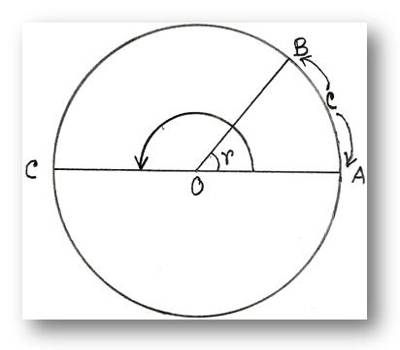Radian is a unit of angle, the degree being the other unit. When a particle makes or completes one complete revolution, the angle swept by the particle is 360oo or 2π2π radians.

Any quantity is said to be completely defined when it is expressed in terms of magnitude and a unit. A unit is the standard measurement used to compare and measure the similar unknown quantities. However, a radian is defined as the ratio of the arc length of a unit circle to the radius of the unit circle.

Here we will discuss radian is a constant angle. Let O be the center of a circle and radius OR = r. If we take an arc AB = OA = r, then by definition, ∠AOB =1 radian.

Let AO be produced to meet the circle at the point C. Then the length of the arc ABC half the circumference and ∠AOC, the angle at the center subtended by this arc = a straight angle = two right angles.

Now if we take the ratio of the two arcs and that of the two angles, we have

arc AB/arc ABC = r/(1/2 × 2∙π∙r) = 1/ π

∠AOB/∠AOC = 1 radian/2 right anglesBut in geometry, we can show that an arc of a circle is proportional to the angle it subtends at the centre of the circle.

Therefore, ∠AOB/∠AOC = arc AB/arc ABC

or, 1 radian/2 right angles = 1/π

Therefore, 1 radian = 2/π right angles

This is constant as both 2 right angles and π are constants.

The approximate value of π is taken as 22/7 for calculation.

Information Source: